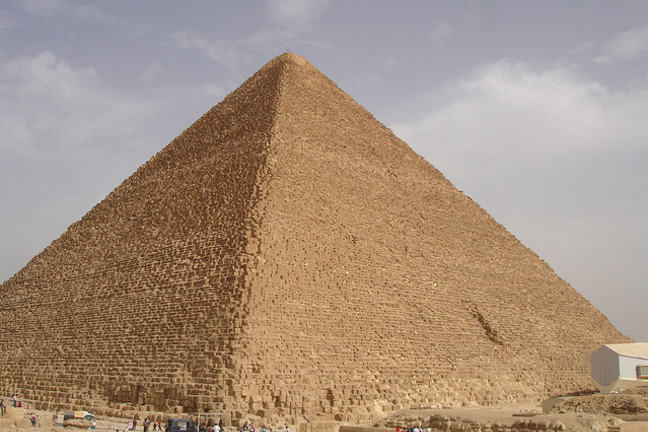## Want to keep learning?

This content is taken from the UNSW Sydney's online course, Maths for Humans: Linear, Quadratic & Inverse Relations. Join the course to learn more.
1.4

## UNSW Sydney# Thales and the measurement of the Great Pyramid

The historical roots of direct proportions probably lie in ancient bartering: I will trade my cow for sixteen of your goats. Mathematically though, the subject begins with a remarkable Greek named Thales, and his attempt to measure the height of the Great Pyramid is.

In this step, we

• learn about the relation between similar triangles, slopes of lines and direct proportions

• find out how high the Great Pyramid is

## Thales and the Great Pyramid of Cheops

Thales was arguably the founder of the great ancient Greek tradition of mathematics and a teacher of Pythagoras. He lived around 600 BC and travelled widely in the Mediterranean region, including to Egypt, which was long the dominant historical power in that area. Egypt of course is home to the great pyramids at Giza, which were already ancient, having been built more than two thousand years previously.

Thales asked the Egyptian priests about the height of the Great Pyramid of Cheops, and they did not tell him, so he set about measuring it himself. His method initiated a powerful approach in geometry associated to the name of similar triangles. While we do not know exactly what method Thales used, similar triangles would certainly have played a key role.

One possibility is that he could have stuck a stick in the ground and waited for the moment in the day when the shadow of that stick was equal in length to the stick. At that precise time, the length of the pyramid’s shadow should be equal to the pyramid’s height.

But how did Thales know how long the shadow was? He could measure the observable shadow, and add half the base size of the pyramid! His conclusion was that the height was about $$\normalsize{481}$$ feet, or $$\normalsize{146.5}$$ m.© “Thales Theorem 6” by Dake/Wikimedia Commons CC BY SA 3.0

The Great Pyramid was the world’s tallest building for over 3,800 years, until the building of the Lincoln cathedral in the 1300s. It has a base which is pretty well exactly a square. In the first few centuries it was covered with some special white stones, but these were eventually removed. So it originally would have been a bit taller than now.

## The slope of a line

There is a more general principle here: that if the rays of the sun are considered at a fixed time of day, then they are all essentially parallel. So the ratio of the height of a stick to the length of its shadow will be the same for all sticks (or pyramids) independent of their height.

This common quantity of

$\Large{\text{height of stick} \over{\text{length of shadow}}}$

is called the slope of the line made by the sun’s rays. Another word for slope is gradient. Often times the gradient is expressed as a percentage. Thus a slope of $$\normalsize{0.3}$$ is often called a gradient of $$\normalsize{30\%}$$.

Q1 (E): What is the slope of Thales’ line when the height of the stick equals the length of the shadow?

Q2 (M): If the perimeter of the base of the Great Pyramid is $$\normalsize{3023}$$ feet, what is the slope of one of its sides?

A1. If height and length are equal, then they are directly proportional with constant of proportionality $$1$$. Equivalently, you can calculate this directly:
${\Large \frac{\text{height of stick}}{\text{length of shadow}} = \frac{\text{length of shadow}}{\text{length of shadow}} = 1 }.$
A2. We have learnt that the height of the Great Pyramid is about $$\normalsize{481}$$ feet. We are told that the perimeter is about $$\normalsize{3023}$$ feet. Hence each side has length $$\normalsize{755.75}$$ and half of that is $$\normalsize{377.875}$$ feet. So we are talking about the slope of a side of a triangle, where the height is $$\normalsize{481}$$ feet and the base is $$\normalsize{377.875}$$ feet. The slope is then $$\normalsize{\frac{481}{377.875}}$$ which is about $$\normalsize{1.27}$$.